One question we often get asked is how to move or copy files based off a certain time criteria, say copying files that are older than 24 hours into an archive folder.  With Automate, this is done by using the File System Action.  As you can see from Figure 1, there are multiple times (Created, Modified and Accessed) to consider when looking at file properties. In these instances, Automate uses the Last Modified time for all of its calculations.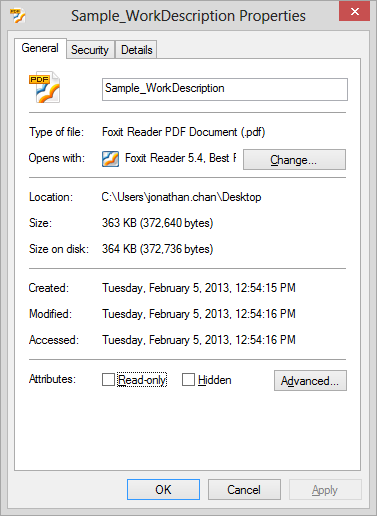Figure 1

Let’s start by selecting the File System Action from the Action Library.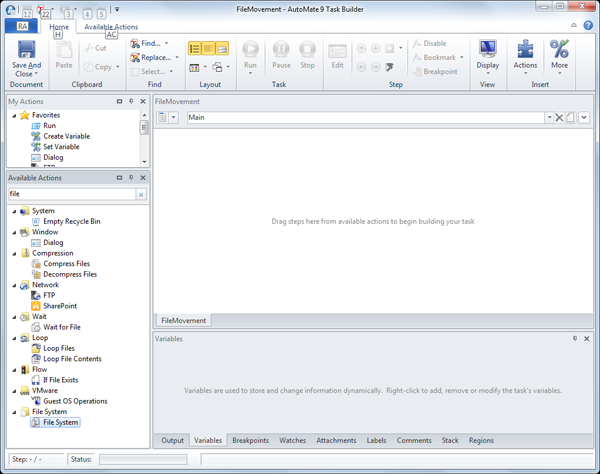Figure 2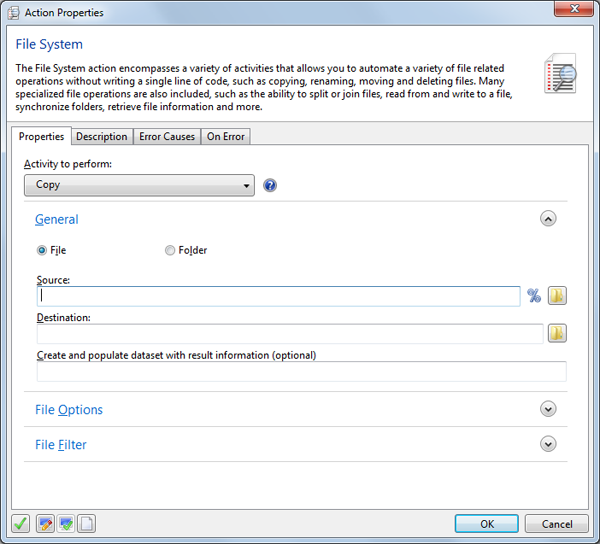Figure 3

Once we have selected the above property, we need to specify the source and destination.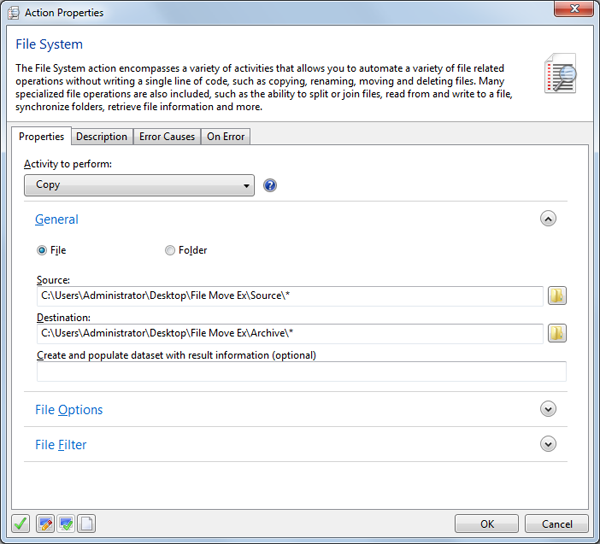Figure 4

After the Source and Destination paths have been entered, expand the File Options Tab. This is to define that only files should be moved if they are older than 24 hours.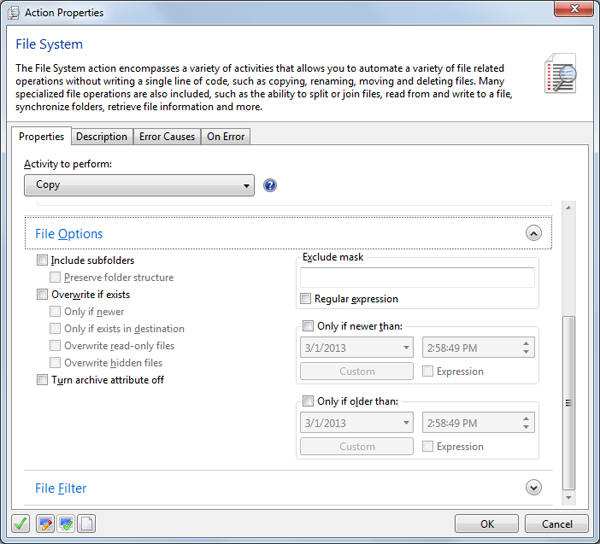Figure 5

Next we’re going to check the “Only if older than” checkbox (see Figure 6).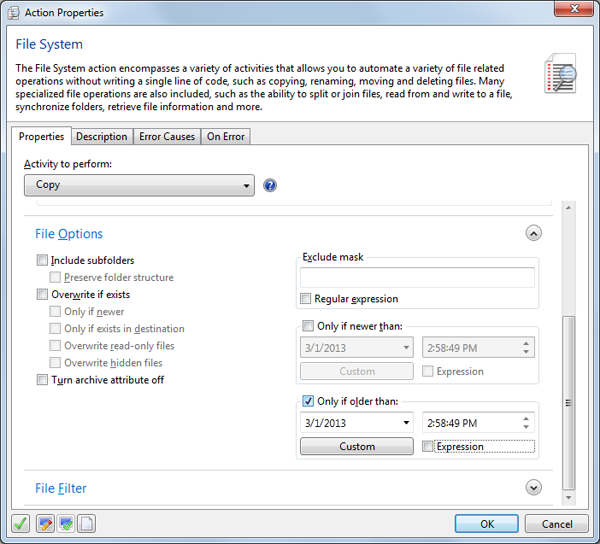Figure 6

Now we need to specify we only want files that are older than 24 hours.  The first is to click the custom button which will give you some of the standard date intervals such as last/next minute/hour/day/month etc. We’re going to select the “Last 24 Hours” (see Figure 7).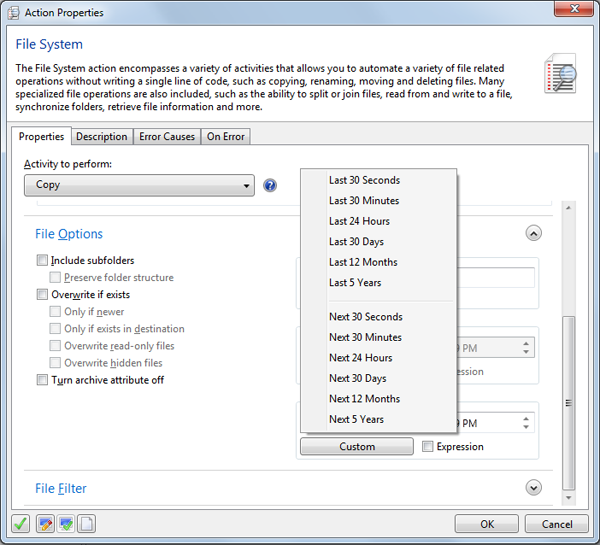Figure 7

Once this is complete, notice how there is some text located in the “Only if older than” box.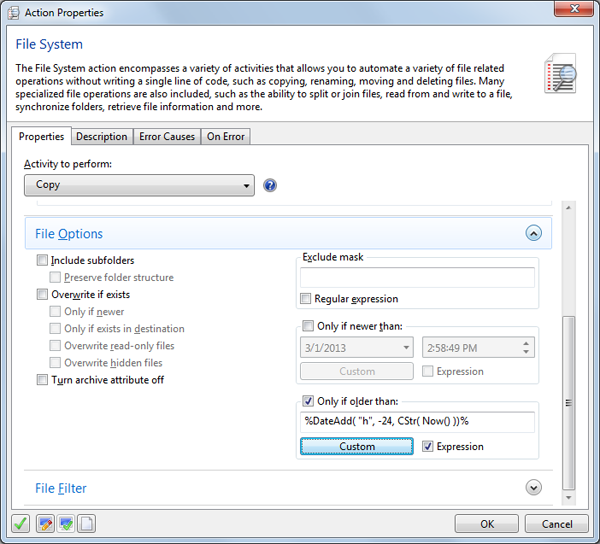Figure 8

%DateAdd( "h", -24, CStr( Now() ))% is the expression that is generated inside the textbox.

After the DateAdd function, there are three comma-separated items: “h”, -24, CStr(Now()). These are what we call parameters. Looking at the documentation, it tells us the structure of these items:

Interval

The first item, string values, indicates which kind of interval to add. Looking at the documentation again, the following characters correspond to specific intervals:

 yyyy Year q Quarter m Month y Day of year d Day w Weekday ww Week h Hour n Minute s Second

Number

In this example the “h” corresponds to hours. If your interval was every quarter, you would use “q”. If you wanted it to be days, you would use “d”, etc.

This second item indicates how many intervals to add, and how your first parameter (interval) is shifted. If the number is positive, then it is in the future. Likewise, a negative number means that it is in the past. The number is -24 in the example. Since the interval is “h” we know that the specified time is shifted 24 hours in the past.

Dateexpr

The last item is Dateexpr, which is the reference date. It calculates the new data relative to this date value. If this value is Null, then Null is returned.  In the example, this value is CStr( Now () ).

This statement is doing multiple things at the same time. The Now() is getting the current date and time from the system. The CStr that is wrapped around it is doing what is called typecasting. Typecasting can be thought of as translating a language. Let’s say I received a document in Japanese, but I can only understand English.  In order to understand and use the document, I will need to first translate it from Japanese before working with it. The same logic applies in this situation. The input that is required may sometimes not come with the correct “translation.” Therefore, it needs to be “translated” before it can be used. In this case, the parameter is expected in a string type—thus we use the CStr() to convert the current date/time into a format that the machine understands.